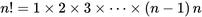# 阶乘也很有趣：从阶乘到伽玛函数再到非整数阶乘https://en.wikipedia.org/wiki/Factorial

100！ 是一个158位的整数

100！ 这么大的数字是怎么算出来的？

import math

digit_num =0.0

for i in range (100):

digit_num = math.log10(i 1)

print(digit_num)

157.97000365471575（约），即

Stirling()公式——Stirling的近似

Stirling公式与阶乘曲线的比较

p>

n!, n=0..4

n!, n=0..6

n!, n=0..10

p>

n!, n=0..10

n!, n=0..10

n! p>

gamma函数与实数域层次的关系

p>

p>

(cosx isinx)的图！